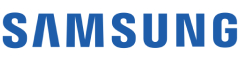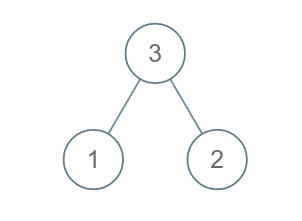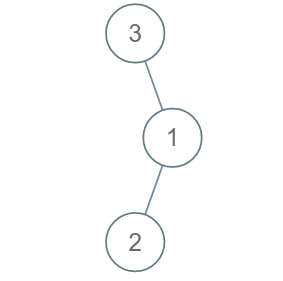New update is available. Click here to update.
Topics

# Height of Binary Tree

Easy0/40
Average time to solve is 15m+13 more companies

## Problem statement

The height of a tree is equal to the number of nodes on the longest path from the root to a leaf.

You are given an arbitrary binary tree consisting of 'n' nodes where each node is associated with a certain value.

Find out the height of the tree.

Example :
``````Input: Let the binary tree be:
````````````Output: 2

Explanation: The root node is 3, and the leaf nodes are 1 and 2.

There are two nodes visited when traversing from 3 to 1.
There are two nodes visited when traversing from 3 to 2.

Therefore the height of the binary tree is 2.
``````
Detailed explanation ( Input/output format, Notes, Images )
Sample Input 1:
``````3 1 2 -1 -1 -1 -1
``````

Sample Output 1:
``````2
``````

Explanation for sample input 1:
``````The given tree is:
````````````The root node is 3, and the leaf nodes are 1 and 2.

There are two nodes visited when traversing from 3 to 1.
There are two nodes visited when traversing from 3 to 2.

Therefore the height of the binary tree is 2.
``````

Sample Input 2:
``````3 -1 1 2 -1 -1 -1
``````

Sample Output 2:
``````3
``````

Explanation of sample input 2 :
``````The given tree is:
````````````The root node is 3, and there is only one leaf node, which is 2.

All three nodes are visited while traversing from 3 to 2.

Therefore the height of the binary tree is 3.
``````

Sample Input 3:
``````2 -1 -1
``````

Sample Output 3:
``````1
``````

Expected time complexity :
``````The expected time complexity is O(n).
``````

Constraints :
``````1 <= 'n' <= 10000

Time Limit: 1 second
``````Console